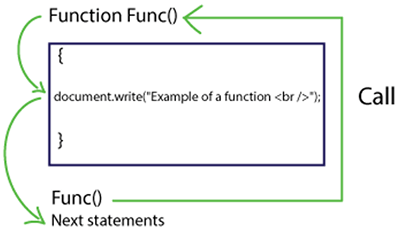JavaScript Functions || JavaScript Functions Examples# JavaScript Functions

2511

Let's go through one of the fundamental concepts of JavaScript named as "Function" contains a block of statements fetching certain inputs, performing computation tasks on them and after that returns some output. There is thousands of documentation found across internet explaining functions; we are here to illustrate function through basic examples.

Objective of Functions

Code reusability

Applications

Function is implemented on those programs where block of codes required to be used multiple times. One can easily create a function with certain lines of code which further can be called and executed whenever required.

Let's prelude function:

Function, we can define it as a subprogram created for performing specific tasks, it can be looping, and conditional checking may be some process based on some input values.

Every function must have some suitable name.

Now let's explore the syntax of a function. It consists of function name, function keyword and required statements as written below:

```function functionName()
{
Blocks of statements relevant to a task
}```

Rules of function

To perform any element of any programming language, there must be some protocols that programmers need to follow during implementation. Similar to other concepts like variable and identifiers, function also contains rules, which we have gathered for you. Rules are almost similar as in variables let's have a look on some rules of functions in JavaScript.

a) Name of function should be initiated with Alphabets only
b) Never use a space
c) Function name may be an inclusive of upper and lower case
d) Underscore and dollars are allowed
e) Keywords can never be a function name for e.g. If, for, break are keywords in JavaScript. It never permits any keywords to be a function name.

Through the syntax and by following rules of function, we have created a function described below:

```function Func()
{
document.write("Example of a function <br />");
}```

After creating a function, the other task is to learn how to execute this function? Will it automatically execute?

No, never

browser will never execute function automatically; we need to call that function. When functions are called for further execution referred as a function invoking.

Function calling is done through func() as above-mentioned.

Process of Function CallingWhenever we have called a function, automatically execution flows jump from Func(); to Func(), then it will executes entire statements present inside them. After successfully completion of entire statements, it will come through the next statement after Func(); will start executing next statements.

Can functions receive any input values?

Suppose I want to perform some calculations such as subtraction of numbers. For subtraction we need to pass at least two values, those two values are called input values. The input values that are passed in a particular function are known as arguments.

In the function, those values are received in some variables; we can call those variables as the parameters in a function.

Through the above explanations, we understood parameter and arguments in JavaScript function.

Now, what is the use of those received variables?

We can utilize those variables in performing any manipulations and calculations.

Let's clear entire concepts on Arguments in any functions

Functions with Arguments

No of passing value should be equals to no of variables existing there. For e.g. subtract (a,b), there exist two variables, then only two values are permitted.

Example

```<html>
<title> Functions in JavaScript!!! </title>
<script type="text/javascript">
{

}
subtract(16,12); //values passed
</script>
<body>
</body>
</html>```

The above-mentioned program will not execute, you can check it through any online compiler because we have just passed two values and received them. After received we are not doing anything with them thus, no output. There is a need to write logic inside curly braces to perform subtraction.

```function subtact(p,q)
{
var z;// local variable declaration to store result
z=p+q;
}```

A simple program to perform a count of Alphabet in name illustrating function arguments

```<html>
<script type="text/javascript">
var count = 0;
function totalAlphabet(name)
{
for (var j=0;j<name.length;j++)
{
if(name[j] >= 'a' && name[j]<='z')
count++;
}
document.write("Hi " + name + "!!! Your name has " + count + " alphabets.");
}
totalAlphabet(myName);
</script>
<body>
</body>
</html>```

Output:

Simple example of Functions:

```<html>
<title>Functions in JavaScript!!!</title>
<script type="text/javascript">
function Func()
{
document.write("Example of a function <br />");
}
Func();
</script>
<body>
</body>
</html>```
Output:
Example of a functionShare:## Get a .COM for just \$6.98

Secure Domain for a Mini Price